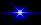# Timer Circuits for Long Delays

Created "July 13 2004"
Updated "March 7 2006"

## Below are Various Circuits using a CD4541B IC. These Chips are capable of Short or VERY LONG Delays Much Longer than a 555

The CD4151B is available from Numerous Manufacturers. The "CD" Prefex is now a "TI" Part Number.

### ** I am still designing up these circuit and will post them as soon as I complete each one.

```"CX" and "RX" determine the Frequency of Oscillation in all these circuits.

Points "A" and "B" are set either Low or High to create Different "Stages" and "Counts".
This along with the selected Frequency can create Very Short to Very Long Delays.

1,000
Frequency = -------------
2.3 * C * R

C = uF
R = K-Ohms
Frequency = Hz

Eg: A 0.22uF Cap and a 12K Resistor = 6.072.

1000
------- = 164.69 Hz
6.072

If pins 12 & 13 are Low, 2N Count = 8182
Therefore N Count = .5 times 8182 = 4091

N Count         4091
Time Delay in Seconds = ----------------- =  ------  = 24.8 Seconds
Frequency in Hz     164.69

If Pins 12 & 13 were both High, 2N Count = 65536
And N Count would = .5 times 65536 = 32768

N Count         32768
Time Delay in Seconds = ----------------- =  -------  = 198.97 Seconds
Frequency in Hz     164.69     or 3.3 minutes

Using a higher value resistor Reduces Frequency
Eg: A 0.22uF Cap and a 220K Resistor = 111.32.

1000
------- = 8,983 Hz
111.32

So if this were the Frequency and if:

If Pins 12 & 13 were both High, 2N Count = 65536
And N Count would = .5 times 65536 = 32768

N Count         32768
Time Delay in Seconds = ----------------- =  -------  = 3,647.8 Seconds
Frequency in Hz      8.983     or 60.8 minutes

Two of these CD4541B's connected as a series circuit with one
driving the other, with the above values, could create a delay of:
60.8 times 60.8 or 3,696.6 Minutes, which is 61.61 Hours.

Or Other ways to Greatly Increase the Set Times.

1) Use a CD4017 "Decade Counter" to give you anywhere from 1 to 10 times this Time.
2) Use a CD4018, "Divide by N Counter". Divisions up to 10.
3) NEED REALLY LONG TIMES, Use a CD4059, "Divide by N Counter",
Allows for Any Division between 3 and 21,327.
Therefore, using this chip and the CD4059 timer of 60.8 minutes
could give 60.8 times 21,327 = 1,296,681.6 minutes or about 21,611.36 hours
***IF YOUR BATTERY DOESN'T DIE FIRST***

As well as making my cicuits below, you may want to get the Data Sheets at "ti.com"
and Experiment for yourself. Like the 555, This is a Very Useful Chip.A Timer that is Useful for Repetitive Triggering
Useful Examples of this circuit: Flashing a Camera for sequential time shots.
Or turning on a Sprinkler every 24 hours.A Timer that has a 50% duty Cycle
Here is a basic circuit using a dip switch to control all the variables of this IC.
Optionally, instead of using the switch, you can take off wires to additional logic circuits.PCB for 50% Timer Above.Picture for 50% Timer Above.Another Timer using the 4541 and a 555 to run a water pump at various cycles.
Added Mar 7 2006, This was a Request from one person.

There are Far Too Many Possibilities to list them all here.